Courses
Courses for Kids
Free study material
Free LIVE classes
More

# How to Find the Coordinates of a Point## Coordinate Points

Coordinates are the two numbers (Cartesian coordinates) or a letter and that point to a specific place on a grid known as a coordinate plane. A coordinate plane has four quadrants and two axes usually represented as x and y axis. The horizontal and vertical distances from the two reference axes are used to describe the position of a point on a coordinate plane.

In the simplest form, there is also formula for coordinates \[y = mx + c\]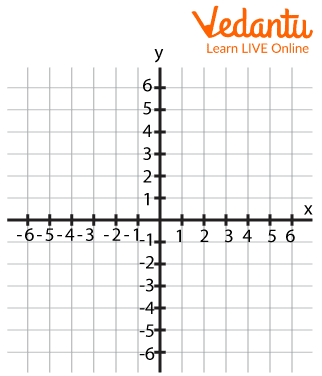Plain Graph Along With four Quadrants

This is quite simple right? we have got to know about coordinates, so now, let’s see about coordinates of points.

## How to Find Coordinates of a Point?

• Go to the coordinate graph with the lines X'OX (in x-axis), Y'OY (in y-axis).

• Identify which quadrant of the graph contains an ordered pair or a point.

• Measure the distance between the point and the x-axis.

• Similarly, to obtain the coordinate value, measure the point's distance from the y-axis.

• There, you will be getting the coordinates of the point.

Not confusing, but let’s see examples which give a clear idea.

### Example 1

In the below given figure, XOX’ and YOY’ are the coordinate axes. Find out the coordinates of point C?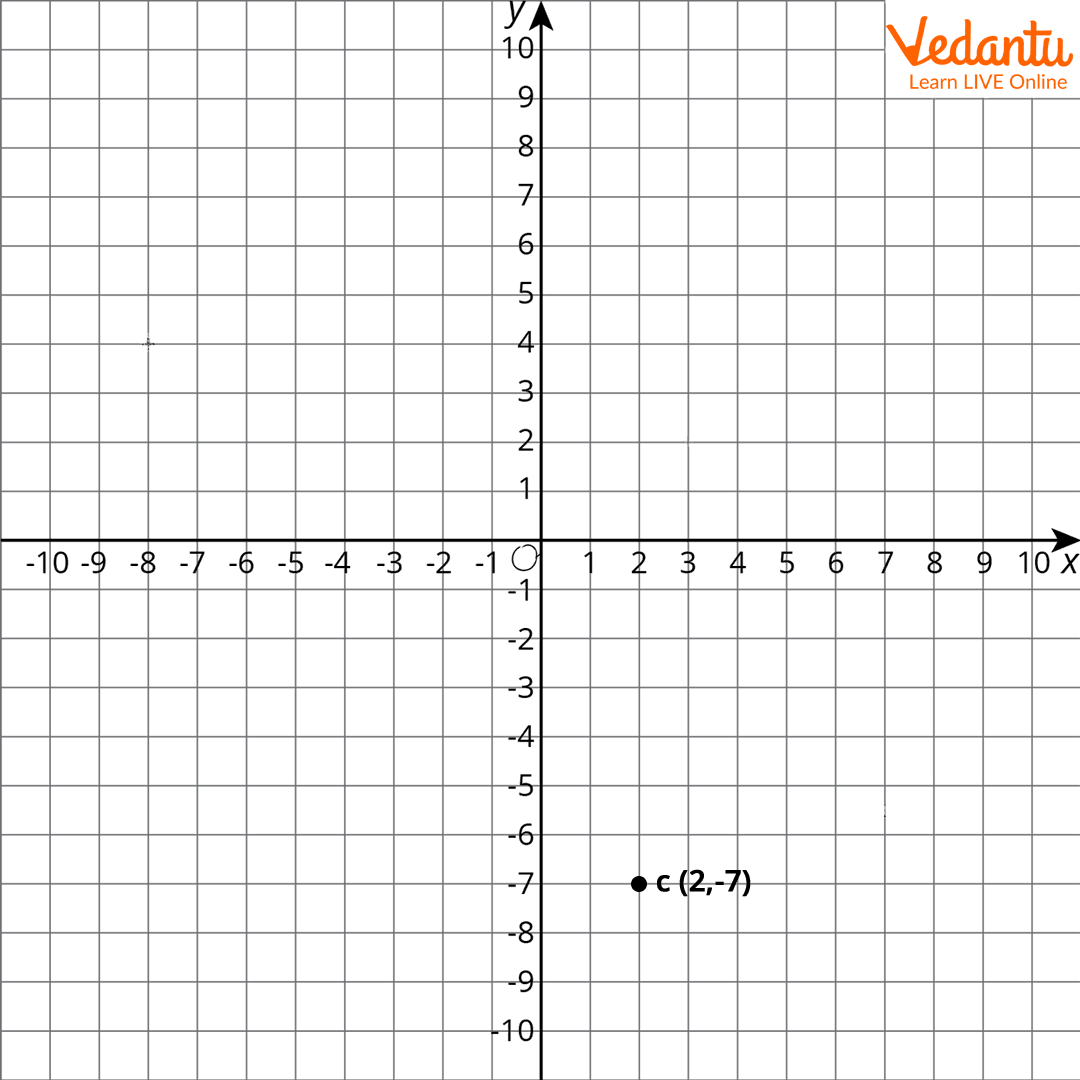Points Marked On a Graph

### Solution :

• Draw straight and perpendicular lines from point C to the x-axis OX and y-axis OY' to identify its position.

• Calculate the distance between the newly obtained point on the x-axis, the origin, and the newly obtained point on the y-axis.

• That location on the x-axis has a value of 2. And the y-axis point has a value of -7.

• As a result, the x coordinate is 2 and the y coordinate is -7.

• The point is in the fourth quadrant since the coordinate is positive and the ordinate is negative.

• ∴ C is the ordered pair (2, -7).

## How to Find Coordinates in Maths?

A series of data that shows an exact position. On graphs, it is typically represented by a pair of numbers: the first number represents the distance along, while the second number represents the distance up or down.

## How to Write Coordinates of A Point?

Coordinates are written as (x, y), which means the x axis point comes first, followed by the y axis point. Some other way may be taught to remember this by saying "along the corridor, up the stairs," which means to follow the x axis first and then the y.

## Conclusion

This topic seems to be of utmost importance at the stage when a student's further study depends  on mathematics in algebra.  A coordinate framework is necessary for defining the exact location of a place in the actual world. To assign geographic locations, a special coordinate system known as the geographical coordinate system is utilised and Coordinates are integers that specify the location of a point or form in a given space (a map or a graph).

### Solved Examples

1: On the Coordinate plane, write the coordinates of points A,B,C,D,E, and F.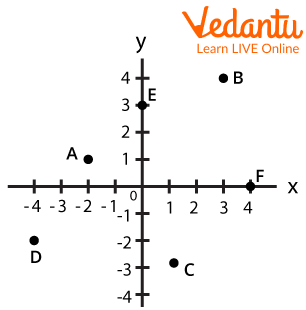Solution :   The provided points' coordinates are: A=(-2,1), B=(3,4), C=(1,-3), D=(-4,-2), E=(0,3), and F= (4,0).

2: On the coordinate plane, write the coordinates of points A, B, C, and D.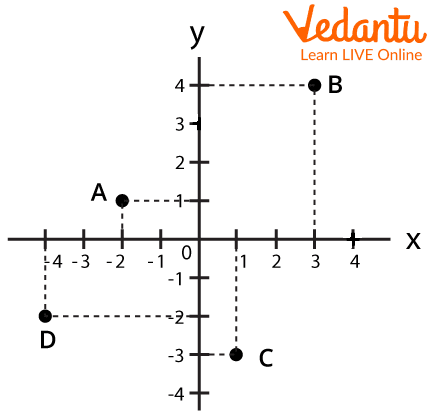Distance Of Different Points On Graph

Solution : The distance between point A and the x axis is one unit, whereas the distance between point A and the y axis is two units. As a result, the coordinates of point A can be represented as A (-2, 1).

(ii) Regarding point B, the point is now 4 units away from the x axis and 3 units away from the y axis. As a result, the coordinates of point B can be expressed as B (3, 4).

(iii) For point C, we can see that it is in the fourth quadrant; thus, the coordinates of point C can be expressed as C (1, -3).

(iv) For point D, we can see that it is in the third quadrant. As a result, the coordinates of the D is D (-4, -2).

3: The point (12,5) is 12 units long and 5 units up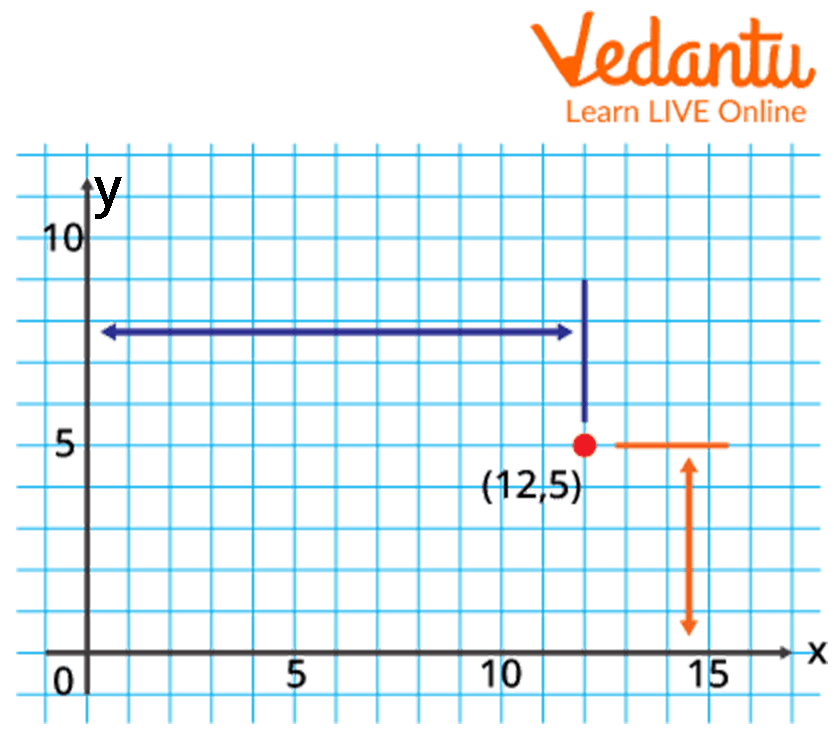Point (12,5) Marked On Graph

Last updated date: 21st May 2023
Total views: 4.5k
Views today: 0.01k

## FAQs on How to Find the Coordinates of a Point

1. How many quadrants are there, what are they?

There are 4 quadrants. A point's coordinates in each quadrant are as follows:

In this case, + represents a positive real number while - represents a negative real number.

2. Find the x and y coordinates of the middle of the line connecting the points (5, -3). (1, 7).

The provided points are (5, -3) and \[({x_2},{y_2})\] respectively (1, 7).

M.P = \[\dfrac{{({x_1} + {x_2})}}{2},\dfrac{{({y_1} + {y_2})}}{2}\] is the midpoint of the line connecting these locations.

M.P = \[\dfrac{{(5 + 1)}}{2},\dfrac{{( - 3 + 7)}}{2}\]

M.P = \[\dfrac{6}{2},\dfrac{4}{2}\]

M.P = \[(3,2)\]

As a result, the x and y coordinates of the midpoint of the line connecting the two locations are (3, 2).

3. A point is located on the x-axis, 8 units away from the y-axis. What are its latitude and longitude? What are the coordinates if it is on the y-axis and -8 units away from the x-axis?

Assume the point is on the x-axis and 8 units away from the y-axis. That is, the point is in the positive x-axis direction, and its y-coordinate is 0. AAs a result, its coordinates are (8, 0).

If the point is -8 units from the x-axis on the y-axis, its x-coordinate must be 0, and the point is in the negative direction of the y-axis.

As a result, its coordinates are (0, -8).

4. Write the coordinates of each of the given figure's points P,Q,R,S,T, and .Image coordinate marked on graph

The coordinates of the needed points are as follows.

P⟹(1,1)

Q⟹(−3,0)

R⟹(−2,−3)

S⟹(2,1)

T⟹(4,−2)

O⟹(0,0)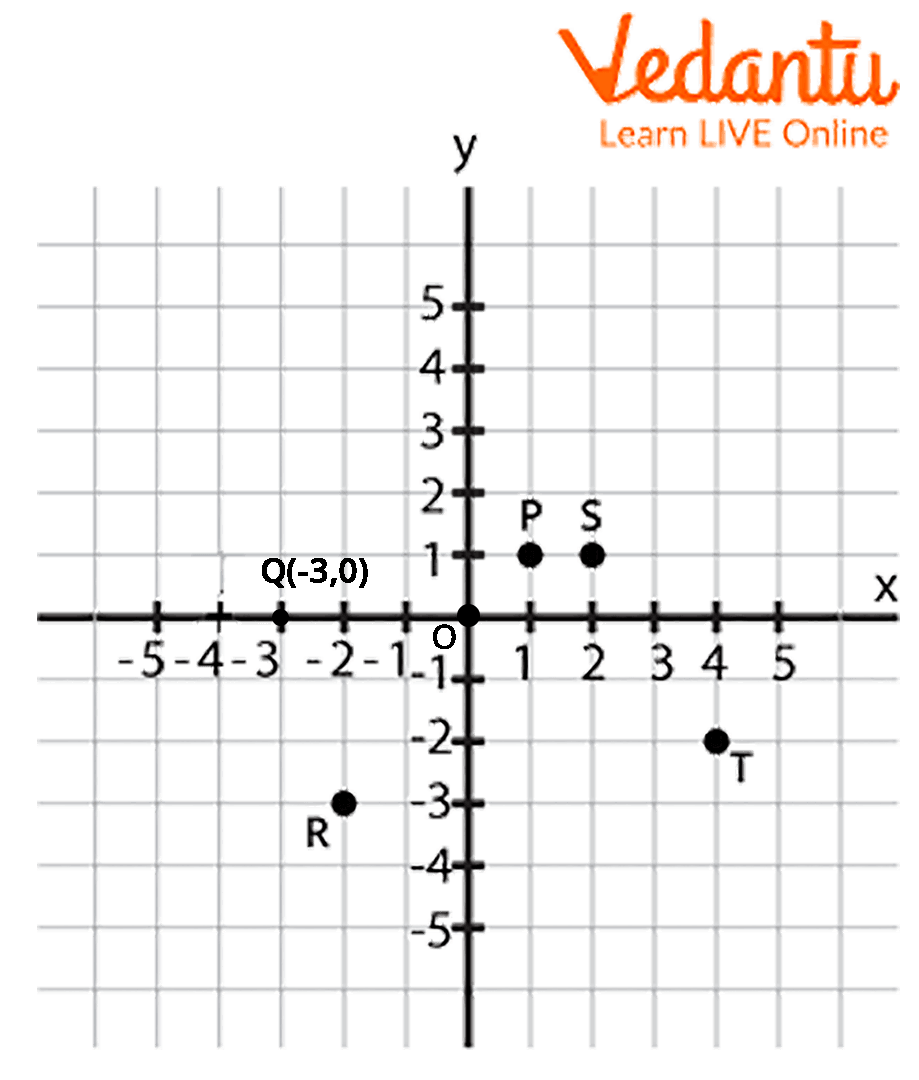Coordinates Of Points P,Q,R,S,T

5. Rectangle ABCD has the following coordinates: A(-5,2), B(8,2), and C(8, -4). Determine D's coordinates.

A graph can assist you solve this challenge. Please see the figure. D must have the coordinates (5,4).Coordinates Of Points A,B,C,D On The Graph